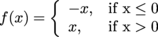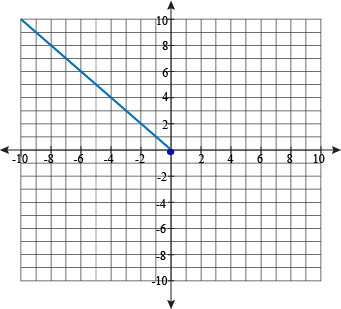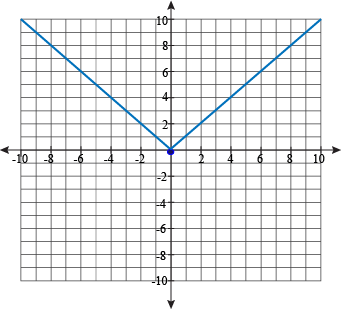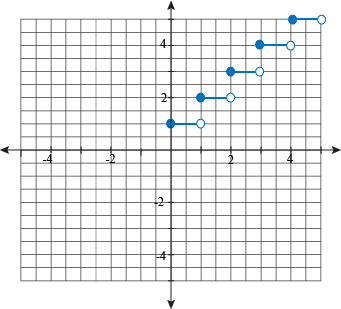Study Guide

# Piecewise Functions

## Piecewise Functions

Wouldn't the name piecewise function be so much more happiness-inducing if the name was peacewise function? We definitely think so. A term comprised of the words "peace" and "wise"? How much better could life get?

Alas, the spelling will always remain piecewise. Why did those prehistoric mathematicians choose that spelling? It's actually a clue as to what the function is.

The word "piecewise" contains the word "piece" in it. As in "piece of pie" or "you want a piece of me?" or "this is the piece of me that you’re never gonna ever take away from me, no." Oh wait, that’s "part of me" isn’t it?

Anyhoo, back to the word "piecewise." It's a function composed of different pieces. That's all.

### How They Work

This is what the most basic piecewise function looks like:Notice how it's defined by two equations. It has two pieces, hence the name.

f(x) = -x

f(x) = x

One equation gives the values of f(x) (you know, y in disguise) when x is less than or equal to 0, and the other gives the values of f(x) when x is greater than 0.

The "if" stuff isn't part of the equation itself, but it tells us which equation to use given the circumstances.

For our piecewise function, if x = -3, we use the first equation and then f(x) = -(-3) = 3. However, if x = 6, we have f(x) = 6.

### How They Look

Now that we know how to decode the "if" statements, we can learn how to graph piecewise functions. Woo?

Don't panic just because you see a foreign notation; think E. T. the friendly alien, not scary Martian from Mars.

We know what the graphs of f(x) = -x and f(x) = x look like, so now all we have to do is put them together using the "if" statements.

First, graph f(x) = -x, but only for when x is less 0, or to the left of (0, 0). We'll draw a solid dot on the point where x = 0, because the inequality sign used is ≤.Then graph f(x) = x, but only for when x is greater than 0, or to the right of (0, 0). The graph will look like a V. Like this:Voilà, that's it.

### Sample Problem

Graph this piecewise function:This has a lot of parts, but it isn't too complicated. The function is a horizontal line at y = 1 when x is between 0 and 1. Then the function goes up to y = 2, 3, 4, and 5 over the same-sized intervals.People call this kind of function a step function, because it looks like a set of steps up a staircase. Mathematicians, with their expensive, highfalutin lifestyles, refused to call it a stair function, because it doesn't look like their marble spiral staircases at all.

Step functions are useful for describing situations when something stays the same a lot of the time, but then jumps up all at once. Like, say, the late fees for keeping The Breakfast Club for two weeks overdue.